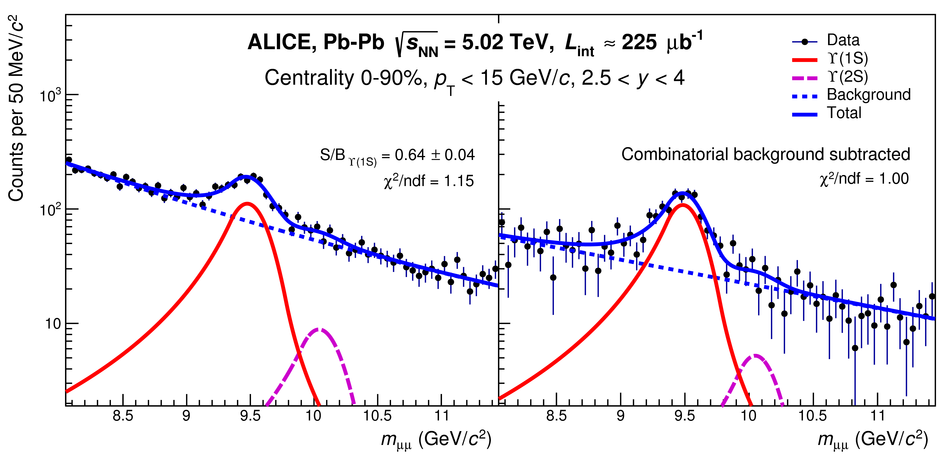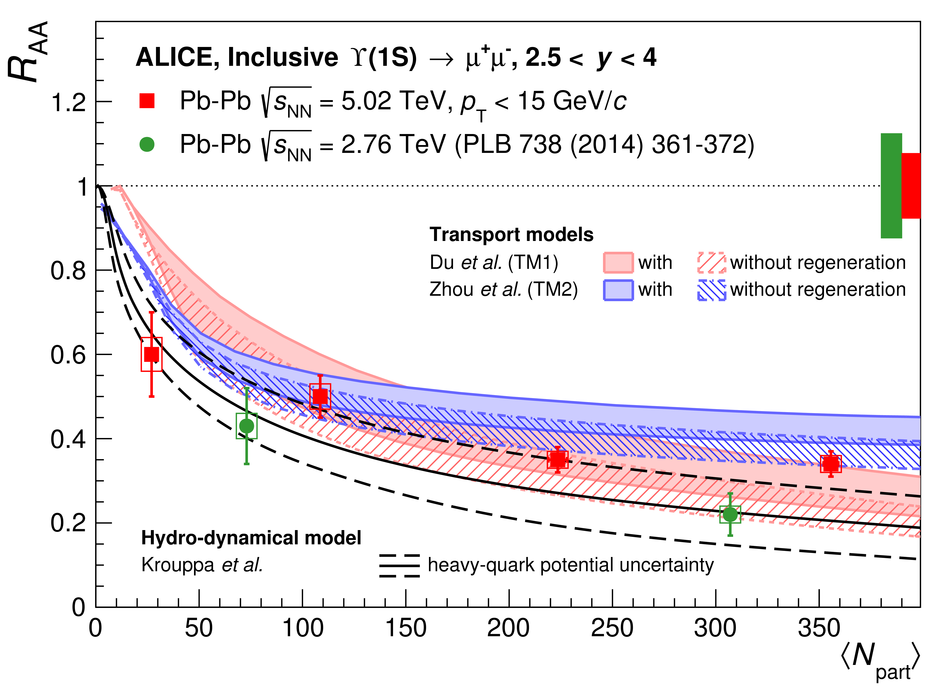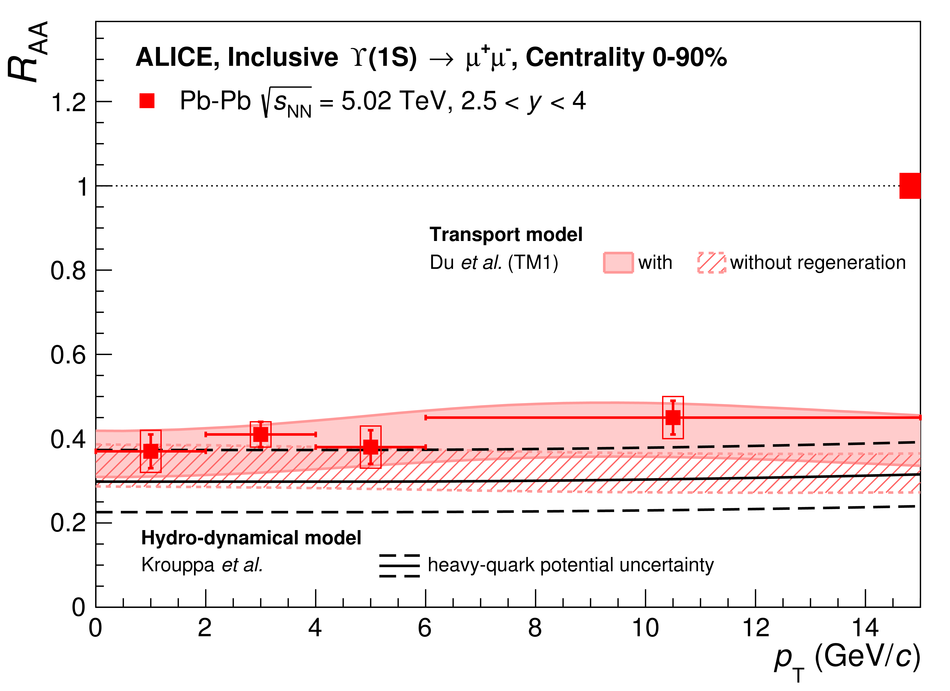# $Υ$ suppression at forward rapidity in Pb-Pb collisions at $\sqrt{s_{\rm NN}}$ = 5.02 TeV

Inclusive $\Upsilon$(1S) and $\Upsilon$(2S) production have been measured in Pb-Pb collisions at the centre-of-mass energy per nucleon-nucleon pair $\sqrt{s_{_{\rm NN}}}=5.02$ TeV, using the ALICE detector at the CERN LHC. The $\Upsilon$ mesons are reconstructed in the centre-of-mass rapidity interval $2.5<~y<~4$ and in the transverse-momentum range $p_{\rm T}<~15$ GeV/$c$, via their decays to muon pairs. In this Letter, we present results on the inclusive $\Upsilon$(1S) nuclear modification factor $R_{\rm AA}$ as a function of collision centrality, transverse momentum and rapidity. The $\Upsilon$(1S) and $\Upsilon$(2S) $R_{\rm AA}$, integrated over the centrality range 0-90%, are $0.37 \pm 0.02 {\rm{(stat)}}\pm 0.03 {\rm{(syst)}}$ and $0.10 \pm 0.04 {\rm{(stat)}}\pm 0.02 {\rm{(syst)}}$, respectively, leading to a ratio $R_{\rm{AA}}^{\Upsilon(\rm2S)}/R_{\rm{AA}}^{\Upsilon(\rm1S)}$ of $0.28\pm0.12\text{(stat)}\pm0.06\text{(syst)}$. The observed $\Upsilon$(1S) suppression increases with the centrality of the collision and no significant variation is observed as a function of transverse momentum and rapidity.

Figures

## Figure 1

 Red and magenta solid lines correspond to \upsis and \upsiss signal functions, respectively. The contribution from \upsisss yield is compatible with zero. Dotted blue lines represent the background (left) and residual background (right), respectively. The sum of the various functions is also shown as a solid blue line.## Figure 2

 Inclusive $\upsis$ $\raa$ as a function of centrality (top), $\pt$ (left) and $y$ (right) at forward rapidity at $\snn=5.02$ TeV. ALICE results at $\snn=2.76$ TeV as a function of centrality and $y$ are shown for comparison~. The vertical error bars and the boxes represent the statistical and uncorrelated systematic uncertainties, respectively. The relative correlated uncertainty is shown as boxes at unity. ALICE $\upsis$ $\raa$ measurements at $\snn=5.02$ TeV are compared to predictions from two transport models and one hydro-dynamical model as a function of centrality (top), $\pt$ (left) and $y$ (right). See text for details on the models.